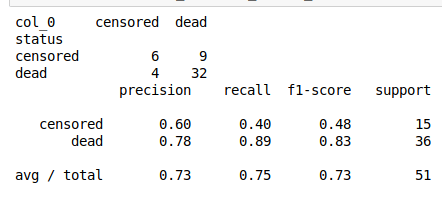# Random Forest Classification with Python

Random forest is a type of machine learning algorithm in which the algorithm makes multiple decision trees that may use different features and subsample to making as many trees as you specify. The trees then vote to determine the class of an example. This approach helps to deal with the high variance that is a problem with making only one decision tree.In this post, we will learn how to develop a random forest model in Python. We will use the cancer dataset from the pydataset module to classify whether a person status is censored or dead based on several independent variables. The steps we need to perform to complete this task are defined below

1. Data preparation
2. Model development and evaluation

Data Preparation

Below are some initial modules we need to complete all of the tasks for this project.

```import pandas as pd
import numpy as np
from pydataset import data
from sklearn.model_selection import train_test_split
from sklearn.model_selection import cross_val_score
from sklearn.ensemble import RandomForestClassifier
from sklearn.metrics import classification_report```

We will now load our dataset “Cancer” and drop any rows that contain NA using the .dropna() function.

```df = data('cancer')
df=df.dropna()```

Next, we need to separate our independent variables from our dependent variable. We will do this by make two datasets. The X dataset will contain all of our independent variables and the y dataset will contain our dependent variable. You can check the documentation for the dataset using the code data(“Cancer”, show_doc=True)

Before we make the y dataset we need to change the numerical values in the status variable to text. Doing this will aid in the interpretation of the results. If you look at the documentation of the dataset you will see that a 1 in the status variable means censored while a 2 means dead. We will change the 1 to censored and the 2 to dead when we make the y dataset. This involves the use of the .replace() function. The code is below.

```X=df[['time','age',"sex","ph.ecog",'ph.karno','pat.karno','meal.cal','wt.loss']]
df['status']=df.status.replace(1,'censored')
y=df['status']```

We can now proceed to model development.

Model Development and Evaluation

We will first make our train and test datasets. We will use a 70/30 split. Next, we initialize the actual random forest classifier. There are many options that can be set. For our purposes, we will set the number of trees to make to 100. Setting the random_state option is similar to setting the seed for the purpose of reproducibility. Below is the code.

```x_train, x_test, y_train, y_test = train_test_split(X, y, test_size=0.3, random_state=0)
h=RandomForestClassifier(n_estimators=100,random_state=1)```

We can now run our modle with the .fit() function and test it with the .pred() function. The code is velow.

```h.fit(x_train,y_train)
y_pred=h.predict(x_test)```

We will now print two tables. The first will provide the raw results for the classification using the .crosstab() function. THe classification_reports function will provide the various metrics used for determining the value of a classification model.

```print(pd.crosstab(y_test,y_pred))
print(classification_report(y_test,y_pred))```Our overall accuracy is about 75%. How good this is depends in context. We are really good at predicting people are dead but have much more trouble with predicting if people are censored.

Conclusion

This post provided an example of using random forest in python. Through the use of a forest of trees, it is possible to get much more accurate results when a comparison is made to a single decision tree. This is one of many reasons for the use of random forest in machine learning.